# Find intersection of Two Linked Lists

Problem Statement: Given the heads of two singly linked-lists headA and headB, return the node at which the two lists intersect. If the two linked lists have no intersection at all, return null.

Examples:

```Example 1:
Input:
List 1 = [1,3,1,2,4], List 2 = [3,2,4]
Output:
2
Explanation: Here, both lists intersecting nodes start from node 2.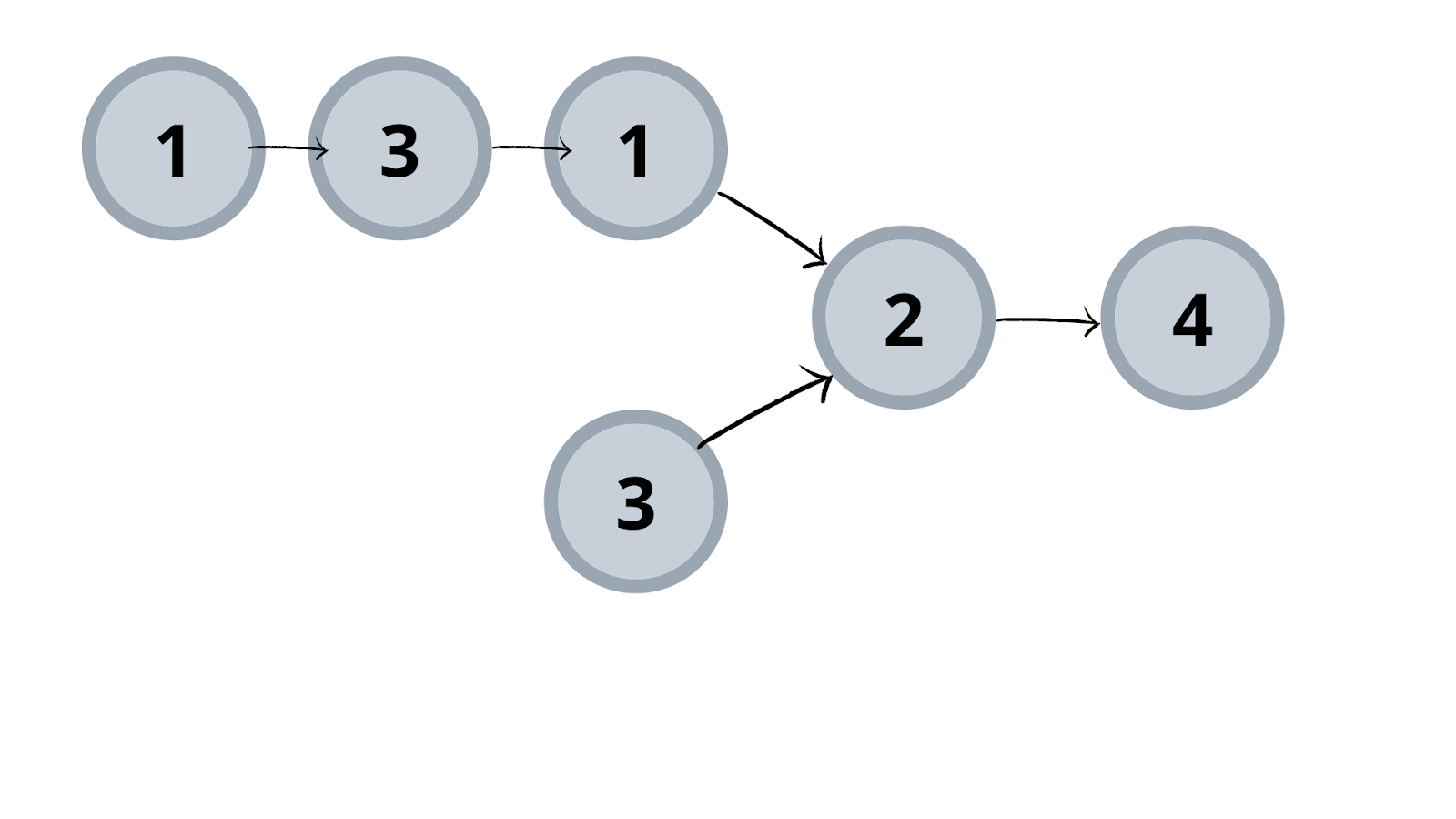```
```Example 2:
Input:
List1 = [1,2,7], List 2 = [2,8,1]
Output:
Null
Explanation: Here, both lists do not intersect and thus no intersection node is present.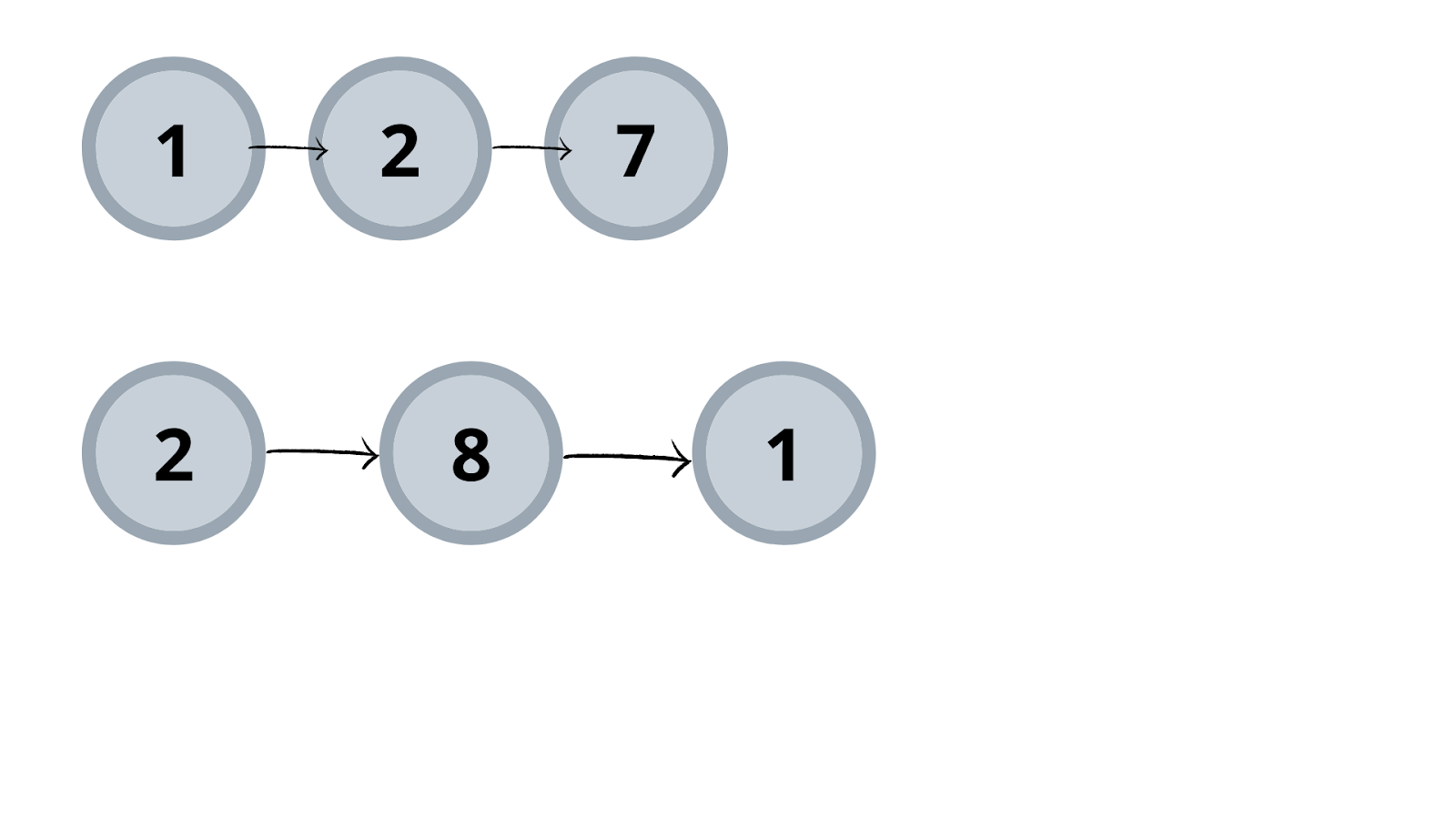```

Solution 1: Brute-Force

Approach: We know intersection means a common attribute present between two entities. Here, we have linked lists as given entities.

What should be the common attribute for two linked lists?

If you believe a common attribute is a node’s value, then think properly! If we take our example 1, there we can see both lists have nodes of value 3. But it is not the first intersection node. So what’s the common attribute?

It is the node itself that is the common attribute. So, the process is as follows:-

• Keep any one of the list to check its node present in the other list. Here, we are choosing the second list for this task.
• Iterate through the other list. Here, it is the first one.
• Check if the both nodes are the same. If yes, we got our first intersection node.
• If not, continue iteration.
• If we did not find an intersection node and completed the entire iteration of the second list, then there is no intersection between the provided lists. Hence, return null.

Dry Run: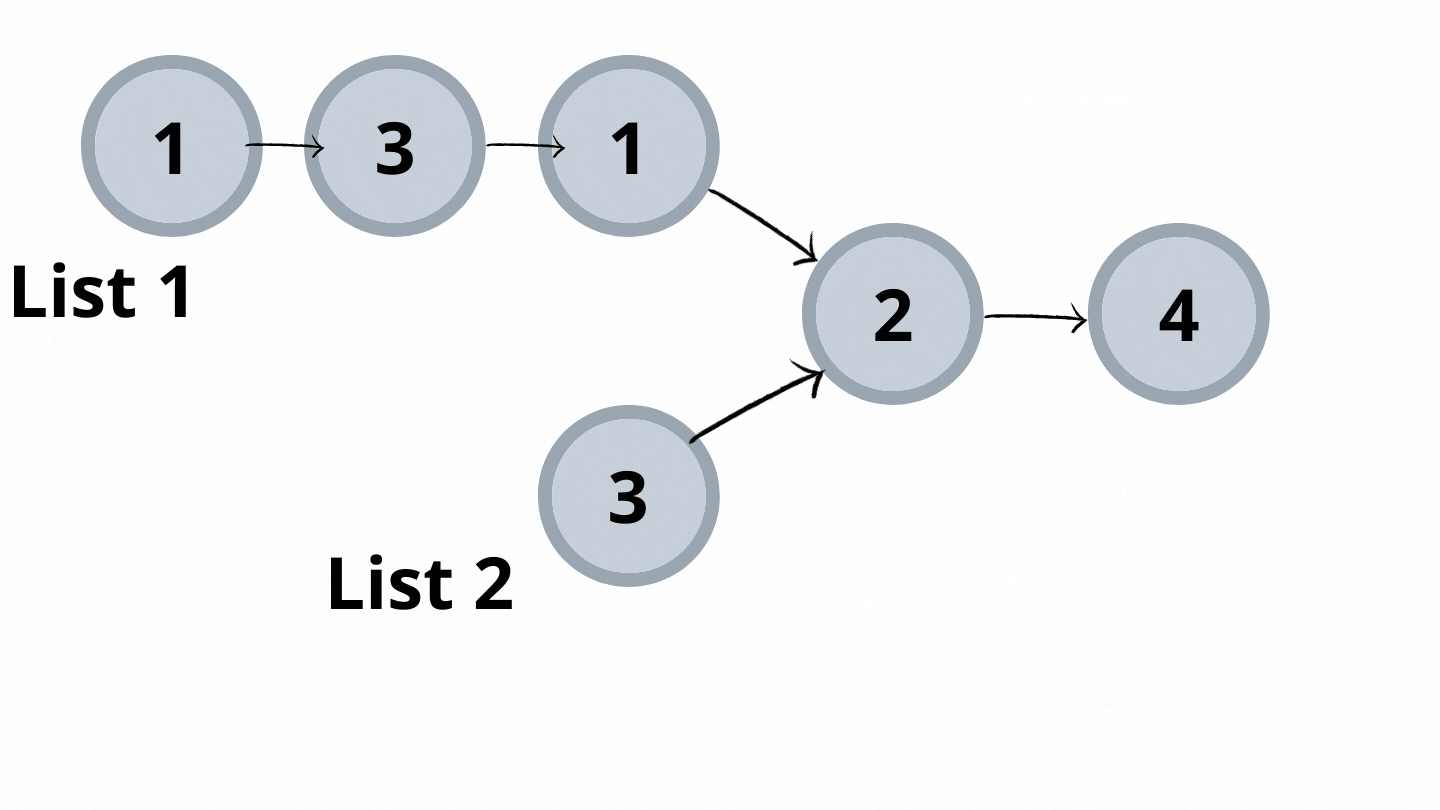Code:

## C++ Code

``````#include<iostream>
using namespace std;

class node {
public:
int num;
node* next;
node(int val) {
num = val;
next = NULL;
}
};
//utility function to insert node at the end of the linked list
void insertNode(node* &head,int val) {
node* newNode = new node(val);

if(head == NULL) {
return;
}

node* temp = head;
while(temp->next != NULL) temp = temp->next;

temp->next = newNode;
return;
}

//utility function to check presence of intersection
while(head2 != NULL) {
node* temp = head1;
while(temp != NULL) {
//if both nodes are same
temp = temp->next;
}
}
//intersection is not present between the lists return null
return NULL;
}

//utility function to print linked list created
void printList(node* head) {
while(head->next != NULL) {
}
}

int main() {
// creation of both lists
node* head = NULL;
node* headSec = NULL;
//printing of the lists
//checking if intersection is present
if(answerNode == NULL )
cout<<"No intersection\n";
else
cout<<"The intersection point is "<<answerNode->num<<endl;
return 0;
}
``````

Output:

List1: 1->3->1->2->4
List2: 3->2->4
The intersection point is 2

Time Complexity: O(m*n)

Reason: For each node in list 2 entire lists 1 are iterated.

Space Complexity: O(1)

Reason: No extra space is used.

## Java Code

``````import java.util.*;
class Node {
int num;
Node next;
Node(int val) {
num = val;
next = null;
}
}
class TUF{
//utility function to insert node at the end of the linked list
static Node insertNode(Node head,int val) {
Node newNode = new Node(val);

if(head == null) {
}

Node temp = head;
while(temp.next != null) temp = temp.next;

temp.next = newNode;
}

//utility function to check presence of intersection
while(head2 != null) {
Node temp = head1;
while(temp != null) {
//if both nodes are same
temp = temp.next;
}
}
//intersection is not present between the lists return null
return null;
}

//utility function to print linked list created
static void printList(Node head) {
while(head.next != null) {
}
}

public static void main(String args[]) {
// creation of both lists
Node head = null;
Node headSec = null;
//printing of the lists
//checking if intersection is present
System.out.println("No intersection\n");
else
System.out.println("The intersection point is "+answerNode.num);

}
}``````

Output:

List1: 1->3->1->2->4
List2: 3->2->4
The intersection point is 2

Time Complexity: O(m*n)

Reason: For each node in list 2 entire lists 1 are iterated.

Space Complexity: O(1)

Reason: No extra space is used.

Solution 2: Hashing

Approach:

Can we improve brute-force time complexity? In brute force, we are basically performing “searching”. We can also perform searches by Hashing. Taking into consideration that hashing process takes O(1) time complexity. So the process is as follows:-

• Iterate through list 1 and hash its node address. Why? (Hint: depends on common attribute we are searching)
• Iterate through list 2 and search the hashed value in the hash table. If found, return node.

Code:

## C++ Code

``````#include<bits/stdc++.h>
using namespace std;

class node {
public:
int num;
node* next;
node(int val) {
num = val;
next = NULL;
}
};
//utility function to insert node at the end of the linked list
void insertNode(node* &head,int val) {
node* newNode = new node(val);

if(head == NULL) {
return;
}

node* temp = head;
while(temp->next != NULL) temp = temp->next;

temp->next = newNode;
return;
}

//utility function to check presence of intersection
unordered_set<node*> st;
while(head1 != NULL) {
}
while(head2 != NULL) {
}
return NULL;

}

//utility function to print linked list created
void printList(node* head) {
while(head->next != NULL) {
}
}

int main() {
// creation of both lists
node* head = NULL;
node* headSec = NULL;
//printing of the lists
//checking if intersection is present
if(answerNode == NULL )
cout<<"No intersection\n";
else
cout<<"The intersection point is "<<answerNode->num<<endl;
return 0;
}
``````

Output:

List1: 1->3->1->2->4
List2: 3->2->4
The intersection point is 2

Time Complexity: O(n+m)

Reason: Iterating through list 1 first takes O(n), then iterating through list 2 takes O(m).

Space Complexity: O(n)

Reason: Storing list 1 node addresses in unordered_set.

## Java Code

``````import java.util.*;
class Node {
int num;
Node next;
Node(int val) {
num = val;
next = null;
}
}
class TUF{
//utility function to insert node at the end of the linked list
static Node insertNode(Node head,int val) {
Node newNode = new Node(val);

if(head == null) {
}

Node temp = head;
while(temp.next != null) temp = temp.next;

temp.next = newNode;
}

//utility function to check presence of intersection
HashSet<Node> st=new HashSet<>();
while(head1 != null) {
}
while(head2 != null) {
}
return null;

}

//utility function to print linked list created
static void printList(Node head) {
while(head.next != null) {
}
}

public static void main(String args[]) {
// creation of both lists
Node head = null;
Node headSec = null;
//printing of the lists
//checking if intersection is present
System.out.println("No intersection\n");
else
System.out.println("The intersection point is "+answerNode.num);

}
}``````

Output:

List1: 1->3->1->2->4
List2: 3->2->4
The intersection point is 2

Time Complexity: O(n+m)

Reason: Iterating through list 1 first takes O(n), then iterating through list 2 takes O(m).

Space Complexity: O(n)

Reason: Storing list 1 node addresses in HashSet.

Solution 3: Difference of length

Approach:

We will reduce the search length. This can be done by searching the length of the shorter linked list. How? Let’s see the process.

• Find length of both the lists.
• Find the positive difference of these lengths.
• Move the dummy pointer of the larger list by difference achieved. This makes our search length reduced to the smaller list length.
• Move both pointers, each pointing two lists, ahead simultaneously if both do not collide.

Dry Run: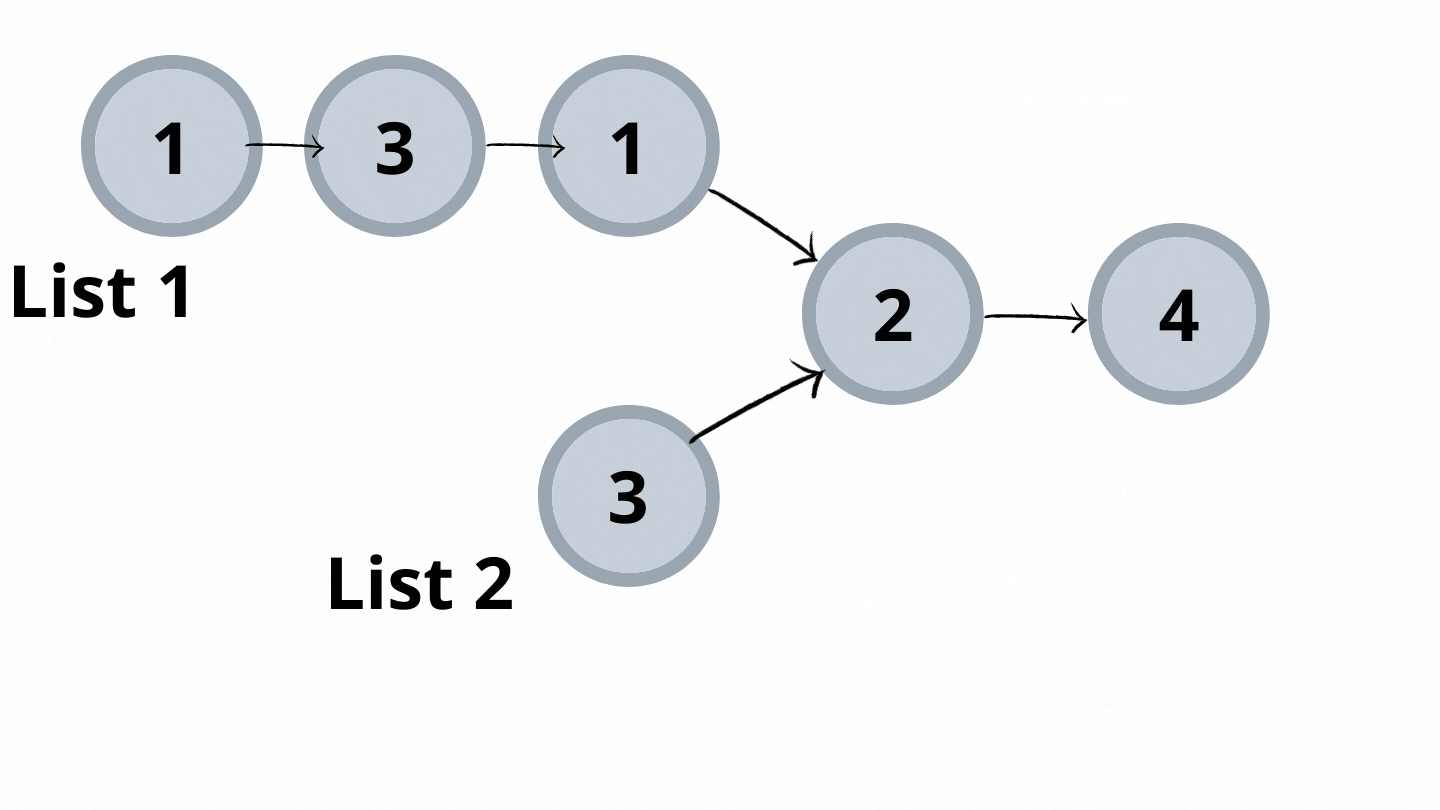Code:

## C++ Code

``````#include<bits/stdc++.h>
using namespace std;

class node {
public:
int num;
node* next;
node(int val) {
num = val;
next = NULL;
}
};
//utility function to insert node at the end of the linked list
void insertNode(node* &head,int val) {
node* newNode = new node(val);

if(head == NULL) {
return;
}

node* temp = head;
while(temp->next != NULL) temp = temp->next;

temp->next = newNode;
return;
}
int len1 = 0,len2 = 0;
while(head1 != NULL || head2 != NULL) {
if(head1 != NULL) {
}
if(head2 != NULL) {
}

}
return len1-len2;//if difference is neg-> length of list2 > length of list1 else vice-versa
}

//utility function to check presence of intersection
if(diff < 0)
else while(diff-- != 0) head1 = head1->next;
while(head1 != NULL) {
}

}

//utility function to print linked list created
void printList(node* head) {
while(head->next != NULL) {
}
}

int main() {
// creation of both lists
node* head = NULL;
node* headSec = NULL;
//printing of the lists
//checking if intersection is present
if(answerNode == NULL )
cout<<"No intersection\n";
else
cout<<"The intersection point is "<<answerNode->num<<endl;
return 0;
}
``````

Output:

List1: 1->3->1->2->4
List2: 3->2->4
The intersection point is 2

Time Complexity:

O(2max(length of list1,length of list2))+O(abs(length of list1-length of list2))+O(min(length of list1,length of list2))

Reason: Finding the length of both lists takes max(length of list1, length of list2) because it is found simultaneously for both of them. Moving the head pointer ahead by a difference of them. The next one is for searching.

Space Complexity: O(1)

Reason: No extra space is used.

## Java Code

``````import java.util.*;
class Node {
int num;
Node next;
Node(int val) {
num = val;
next = null;
}
}
class TUF{
//utility function to insert node at the end of the linked list
static Node insertNode(Node head,int val) {
Node newNode = new Node(val);

if(head == null) {
}

Node temp = head;
while(temp.next != null) temp = temp.next;

temp.next = newNode;
}
int len1 = 0,len2 = 0;
while(head1 != null || head2 != null) {
if(head1 != null) {
}
if(head2 != null) {
}

}
return len1-len2;//if difference is neg-> length of list2 > length of list1 else vice-versa
}
//utility function to check presence of intersection
if(diff < 0)
else while(diff-- != 0) head1 = head1.next;
while(head1 != null) {
}

}

//utility function to print linked list created
static void printList(Node head) {
while(head.next != null) {
}
}

public static void main(String args[]) {
// creation of both lists
Node head = null;
Node headSec = null;
//printing of the lists
//checking if intersection is present
System.out.println("No intersection\n");
else
System.out.println("The intersection point is "+answerNode.num);

}
}``````

Output:

List1: 1->3->1->2->4
List2: 3->2->4
The intersection point is 2

Time Complexity:

O(2max(length of list1,length of list2))+O(abs(length of list1-length of list2))+O(min(length of list1,length of list2))

Reason: Finding the length of both lists takes max(length of list1, length of list2) because it is found simultaneously for both of them. Moving the head pointer ahead by a difference of them. The next one is for searching.

Space Complexity: O(1)

Reason: No extra space is used.

Solution 4: Optimised

Approach:

The difference of length method requires various steps to work on it. Using the same concept of difference of length, a different approach can be implemented. The process is as follows:-

• Take two dummy nodes for each list. Point each to the head of the lists.
• Iterate over them. If anyone becomes null, point them to the head of the opposite lists and continue iterating until they collide.

Dry Run: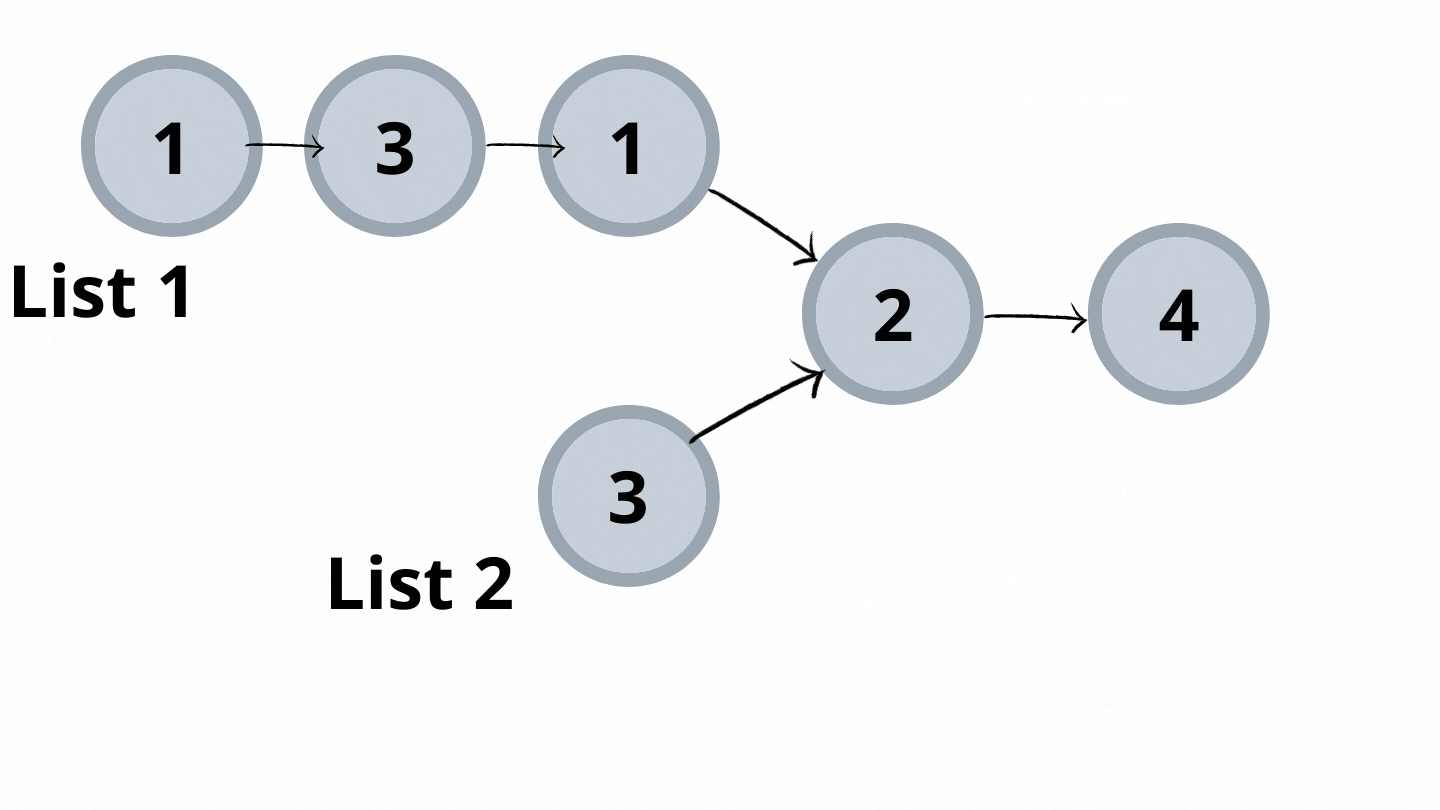Code:

## C++ Code

``````#include<bits/stdc++.h>
using namespace std;

class node {
public:
int num;
node* next;
node(int val) {
num = val;
next = NULL;
}
};
//utility function to insert node at the end of the linked list
void insertNode(node* &head,int val) {
node* newNode = new node(val);

if(head == NULL) {
return;
}

node* temp = head;
while(temp->next != NULL) temp = temp->next;

temp->next = newNode;
return;
}
//utility function to check presence of intersection
node* d1 = head1;
node* d2 = head2;

while(d1 != d2) {
d1 = d1 == NULL? head2:d1->next;
d2 = d2 == NULL? head1:d2->next;
}

return d1;
}

//utility function to print linked list created
void printList(node* head) {
while(head->next != NULL) {
}
}

int main() {
// creation of both lists
node* head = NULL;
node* headSec = NULL;
//printing of the lists
//checking if intersection is present
if(answerNode == NULL )
cout<<"No intersection\n";
else
cout<<"The intersection point is "<<answerNode->num<<endl;
return 0;
}
``````

Output:

List1: 1->3->1->2->4
List2: 3->2->4
The intersection point is 2

Time Complexity: O(2*max(length of list1,length of list2))

Reason: Uses the same concept of difference of lengths of two lists.

Space Complexity: O(1)

Reason: No extra data structure is used

## Java Code

``````import java.util.*;
class Node {
int num;
Node next;
Node(int val) {
num = val;
next = null;
}
}
class TUF{
//utility function to insert node at the end of the linked list
static Node insertNode(Node head,int val) {
Node newNode = new Node(val);

if(head == null) {
}

Node temp = head;
while(temp.next != null) temp = temp.next;

temp.next = newNode;
}
//utility function to check presence of intersection
Node d1 = head1;
Node d2 = head2;

while(d1 != d2) {
d1 = d1 == null? head2:d1.next;
d2 = d2 == null? head1:d2.next;
}

return d1;
}

//utility function to print linked list created
static void printList(Node head) {
while(head.next != null) {
}
}

public static void main(String args[]) {
// creation of both lists
Node head = null;
Node headSec = null;
//printing of the lists
//checking if intersection is present
System.out.println("No intersection\n");
else
System.out.println("The intersection point is "+answerNode.num);

}
}``````

Output:

List1: 1->3->1->2->4
List2: 3->2->4
The intersection point is 2

Time Complexity: O(2*max(length of list1,length of list2))

Reason: Uses the same concept of difference of lengths of two lists.

Space Complexity: O(1)

Reason: No extra data structure is used

Special thanks to Dewanshi Paul for contributing to this article on takeUforward. If you also wish to share your knowledge with the takeUforward fam, please check out this article